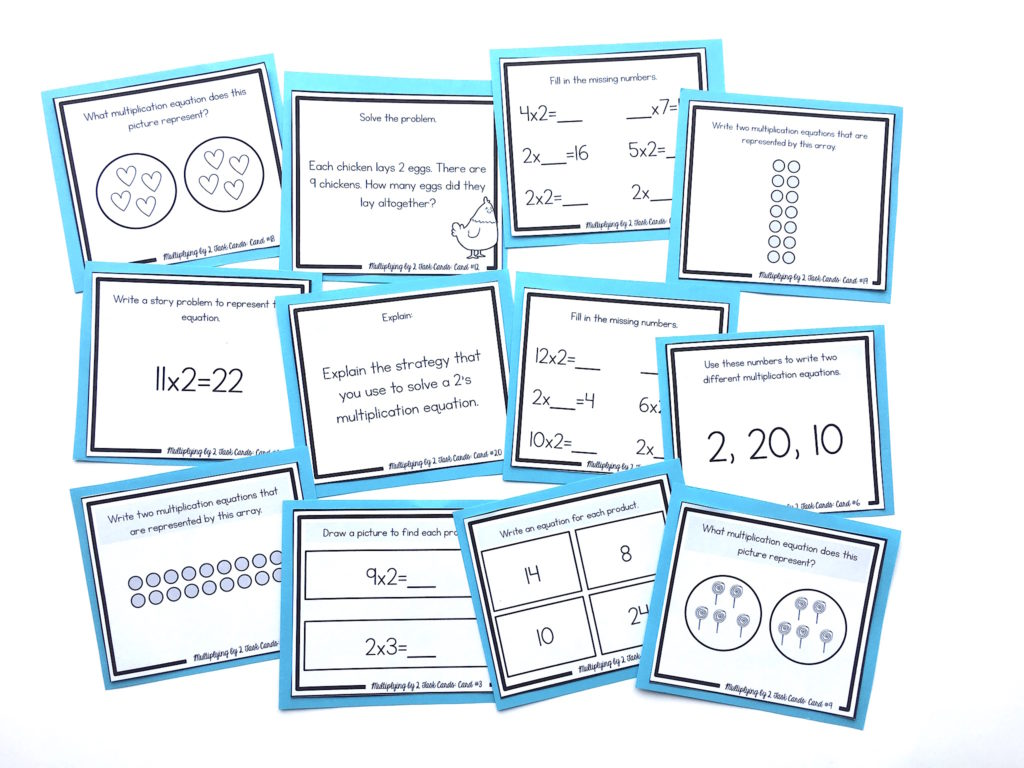# How to Teach the 2’s Multiplication FactsThe 2’s multiplication facts are some of the easiest ones to learn. This is because they can be taught as the addition doubles, which your students should already know.

For example, when you have an equation like 2×3, you can think of this as the double of 3, which is 6.

An equation like 2×7 can be thought of as the double of 7, or 14.

It’s also very important to reinforce the commutative property.

This means that the order of factors does not change the product. For example, if students are faced with an equation like 8×2, they should see the 2 as one of the factors, and know that they can use the 2’s strategy to solve this equation. In this case students can think, “the double of 8.”

NEXT STEPS

Reinforce the 2’s multiplication facts with this set of task cards. Students will learn conceptually through problem-solving, using arrays, strategic thinking, finding missing numbers, skip-counting, picture representations, and more:OR FIND THE FULL BUNDLE OF MULTIPLICATION TASK CARDS HERE.